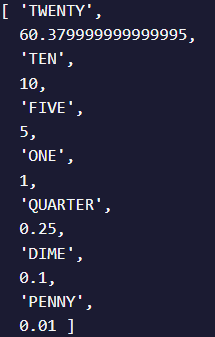# Cash register problem, cant do it

Tell us what’s happening:is it too hard or am i dumb

I’m trying to switch to the next currency when the register runs out of that money, but it doesn’t work and i don’t why. And the first one is float couldn’t fix that either.

every kind of help would be much appreciated, thanks in advance

``````
function checkCashRegister(price, cash, cid) {
let change = cash - price;
let status;
let currency = [0.01, 0.05, 0.1, 0.25, 1, 5, 10, 20, 100];
let arr = [];

let money = 0;
for(let i=0; i < cid.length; i++){
money += cid[i];
}

if(change > money) return {status: "INSUFFICIENT_FUNDS", change: []};

for(let i = currency.length - 1; i >= 0; i--){
let result = 0;
let counter = 0;

while(currency[i] <= change){   // something is wrong here, i think
change -= currency[i];
change = change.toFixed(2);
cid[i] -= currency[i];

if(cid[i] < 0){
continue;
} else {
result += currency[i];
}

if(status === undefined) status = "OPEN";

if(counter === 0){
arr.push(cid[i], result);
} else {
arr += currency[i];
}
counter++;
}

}

let final = {status: status, change: [arr]}
console.log(arr)
return final;
}

checkCashRegister(3.26, 100, [["PENNY", 1.01], ["NICKEL", 2.05], ["DIME", 3.1], ["QUARTER", 4.25], ["ONE", 90], ["FIVE", 55], ["TEN", 20], ["TWENTY", 60], ["ONE HUNDRED", 100]]);
``````
``````  **Your browser information:**
``````

User Agent is: `Mozilla/5.0 (Windows NT 10.0; Win64; x64) AppleWebKit/537.36 (KHTML, like Gecko) Chrome/100.0.4896.75 Safari/537.36`

Challenge: Cash Register

is it too hard or am i dumb

This is definitely hard for learners. I’d already had some coding experience and I remember struggling with this.

When in doubt, I like to log out inside the function to see what is happening:

``````function checkCashRegister(price, cash, cid) {
let change = cash - price;
let status;
let currency = [0.01, 0.05, 0.1, 0.25, 1, 5, 10, 20, 100];
let arr = [];

let money = 0;
for(let i=0; i < cid.length; i++){
money += cid[i];
}

if(change > money) return {status: "INSUFFICIENT_FUNDS", change: []};

for(let i = currency.length - 1; i >= 0; i--){
console.log('\n* currency', currency[i])
let result = 0;
let counter = 0;

while(currency[i] <= change){   // something is wrong here, i think
console.log('\n*** start of while, change', change)
change -= currency[i];
change = change.toFixed(2);
cid[i] -= currency[i];

if(cid[i] < 0){
continue;
} else {
result += currency[i];
}

if(status === undefined) status = "OPEN";

if(counter === 0){
console.log('*** counter is 0, counter is', counter)
arr.push(cid[i], result);
} else {
console.log('*** counter is not 0, counter is', counter)
arr += currency[i];
}
console.log('*** arr after', arr)
counter++;
console.log('*** end of while, change', change)
}

}

let final = {status: status, change: [arr]}
console.log('final', final)
return final;
}
``````

I would trace through and try to figure out what is happening when and why. I also don’t think that that is the correct shape of data for your final answer - I think they have another layer of arrays.

1 Like

Not sure if there is a specific output format expected, but here’s one way of solving the problem:

``````const REGISTER = [
[0.01, 10],
[0.05, 10],
[0.1, 50],
[0.2, 20],
[0.5, 10],
[1, 50],
[5, 20],
[10, 10],
[20, 2],
[50, 4],
[100, 1]
];

function getChange(cost, cash) {
let changes = {};
if (cash < cost) {
return "Not enough cash!";
}

const totalChange = cash - cost;
let change = totalChange;

const registerDesc = REGISTER.sort((a, b) => {
if (a > b) return -1;
if (a < b) return 1;
return 0;
});

for (const [deno] of registerDesc) {
if (change === 0) continue;

const bills = Math.floor(change / deno);
if (bills > 0) {
changes[[deno]] = bills;
}
change = change % deno;
}

console.log(
`For a purchase of \$\${cost}, I paid \$\${cash}\nand I received a change of \$\${totalChange} in the following denominations:`
);

for (const [k, v] of Object.entries(changes)) {
console.log(`\${v} x \$\${k}`);
}
}

getChange(251, 500);
``````

Btw, I intentionally left out the `Insufficient Fund` check

Cheers!

i’ve already solved it. Kevin’s comment was a great debuggeri shall keep that in my mind.